# Dynamics of optimizing Gaussian mixture models¶

This super-simple example illustrates how a Gaussian mixture model behaves with high and low fixed variance (that is, how it collapses or specializes the clusters as in deterministic annealing).

We then examine what happens when we try to optimize the variance together with the means.

• Good news: It's optimal to specialize into low-variance clusters, as expected, and EM or gradient descent will eventually discover this.

• Bad news: Many optimization algorithms are attracted into the neighborhood of a bad saddle point. They then spend most of their time hanging out there before eventually finding the right solution. Or worse, they stop numerically near the saddle point before they get to the right solution.

This bad saddle point is a symmetric solution with high variance. The clusters collapse into a single cluster whose variance matches the sample variance.

• Recommendation: Thus, rather than using the gradient to optimize the variance, it really is a good idea to sweep through different variance values as an outer loop around the optimizer. This actively pushes the system away from the saddle point.

Deterministic annealing (DA) does this. Often DA is sold as a way to (hopefully) escape bad local optima in latent-variable models. But it may also be useful in general for escaping the saddle points of such models, which may not be terminal points for optimization but can greatly slow it down. This super-simple example has no bad local optima, just saddle points.

# Model and log-likelihood function¶

Throughout the investigation, our model is an equal mixture of two univariate Gaussians with symmetric means $\mu,-\mu$ and common variance $\sigma^2$ with $\sigma > 0$. The log-density of the mixture is

In :
# addition in the log domain to avoid underflow.
# This version can add two vectors in parallel.
logadd <- function (a,b) { ifelse(a==-Inf, b,   # The ifelse test avoids the problem that -Inf minus -Inf  NaN.
pmax(a,b) + log1p(exp(pmin(a,b)-pmax(a,b)))) }

In :
# mixture of univariate Gaussians
ldmix <- function (x,mu,sigma) {
sigma <- pmax(0,sigma)   # since an unbounded optimizer might explore sigma < 0, just treat that like 0
# Loses precision:
# log((dnorm(x,mu,pmax(0,sigma))+dnorm(x,-mu,pmax(0,sigma)))/2)
logadd( dnorm(x,mu,sigma,log=T) , dnorm(x,-mu,sigma,log=T) ) - log(2)
}


We'll also assume that the only observed datapoints are at 1 and -1. Then the log-likelihood is

In :
ll <- function (mu,sigma) ldmix(1,mu,sigma)+ldmix(-1,mu,sigma)


Let's visualize that log-likelihood function:

In :
mu <- seq(-2,2,length.out=50)
sigma <- seq(0.5,2,length.out=50)
log_lik <- outer(mu,sigma,Vectorize(ll))
persp(mu, sigma, log_lik, theta=30, phi=30, ticktype="detailed")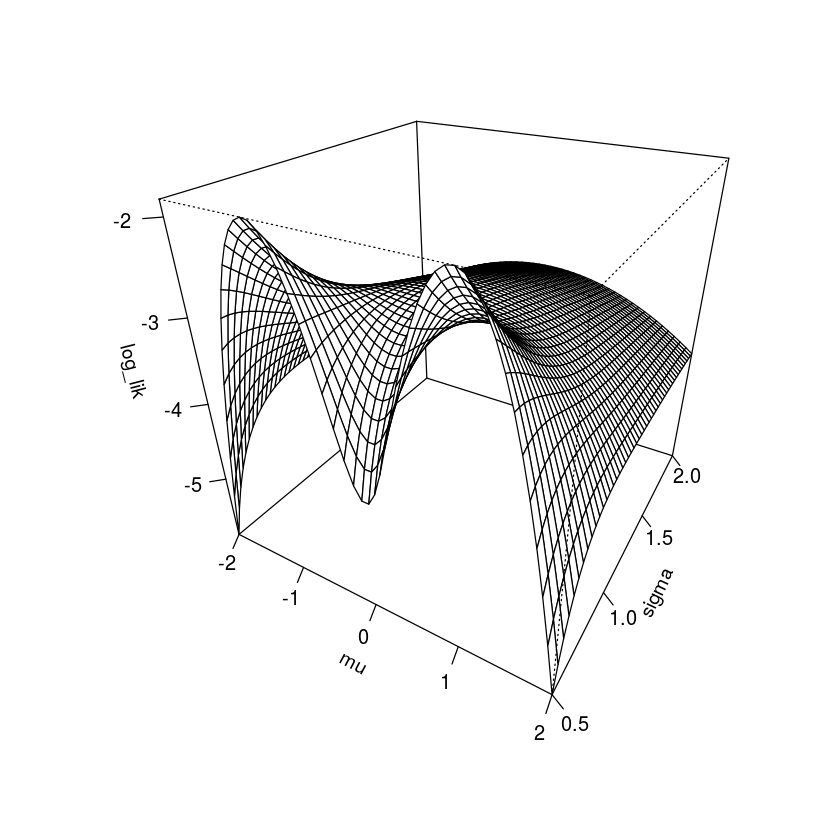At each fixed $\sigma$:

• The symmetry in $\mu$ comes from the fact that we can swap the clusters and get the same likelihood.
• There is an extremum at $\mu=0$,
• which is a local minimum for $\sigma < 1$ (so the clusters like to move apart)
• but a global maximum for $\sigma \geq 1$ (where the clusters like to move together).
• These correspond to low and high temperature in deterministic annealing.
• The phase shift happens at the "critical temperature" where $\sigma = 1$, probably because that's the sample stdev.

We can see that transition by zooming in on $\sigma \approx 1$:

In :
sigma <- seq(0.9,1.1,length.out=50)
log_lik <- outer(mu,sigma,Vectorize(ll))
persp(mu, sigma, log_lik, theta=30, phi=30, ticktype="detailed")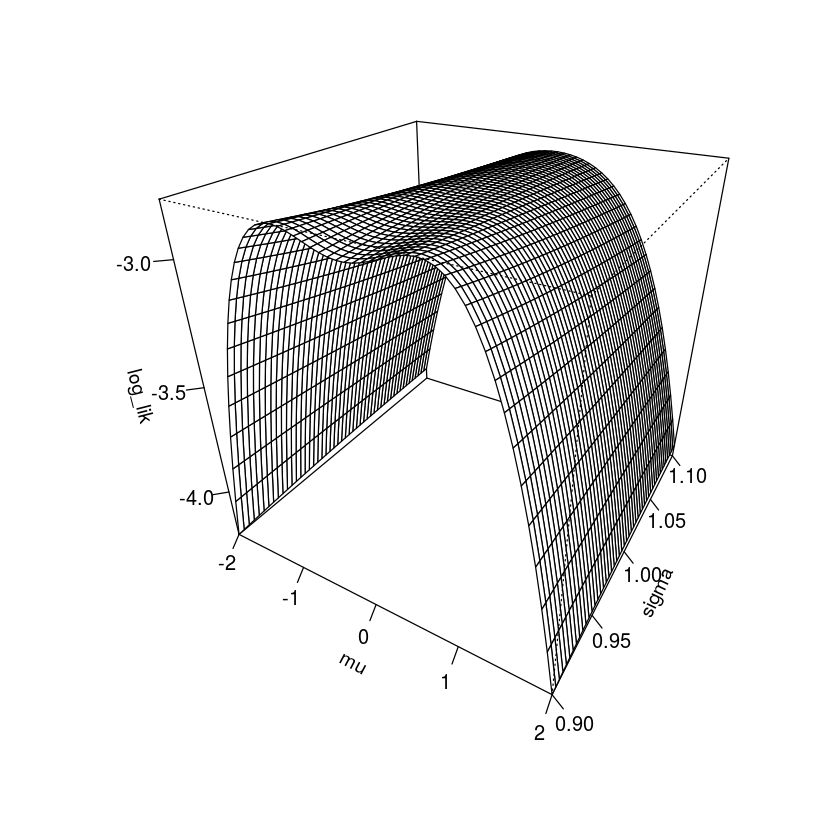If we allow $\sigma$ to vary, however:

• The global maximum puts one cluster at each point, with $\sigma$ as small as possible. (Actually there are two symmetric maxima of this sort.)
• So $\sigma$ wants to become small, allowing the clusters to specialize.

# Alternating optimization¶

But when can we computationally find this maximum? There are are no other local maxima on the whole surface. But finding a global maximum might still be hard! The problem is that $(\mu=0,\sigma=1)$ is a very stable and somewhat attractive saddle point.

Let's see what goes wrong in the case of alternating optimization.

First we hold $\sigma > 1$ fixed while optimizing $\mu$. The argmax is $\mu=0$ (the Gaussian mixture becomes a single Gaussian):

In :
library(repr)
options(repr.plot.width=3, repr.plot.height=3) # smaller plots

In :
plot(function (mu) ll(mu,1.1), xlab="mu", xlim=c(-2,2))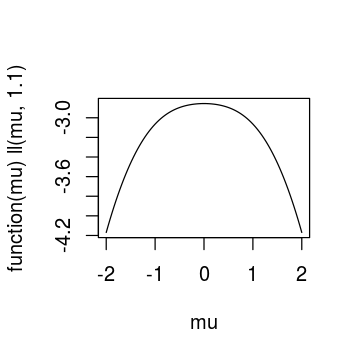Now we hold $\mu=0$ fixed while optimizing $\sigma$. It moves to the sample stdev, namely 1. (In fact, this is true regardless of where $\sigma$ starts.)

In :
plot(function (sigma) ll(0,sigma), xlab="sigma", xlim=c(0.5, 2))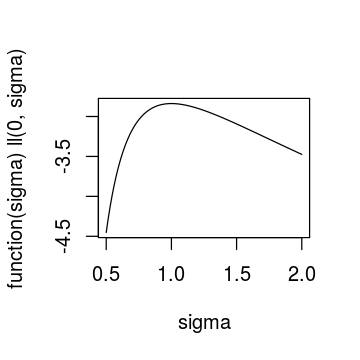We're now stuck at this saddle point $(\mu,\sigma)=(0,1)$: for this $\sigma$, our current $\mu=0$ is already optimal.

In :
plot(function (mu) ll(mu,1), xlab="mu", xlim=c(-2,2))
plot(function (mu) ll(mu,1), xlim=c(-.01,.01))   # zooming in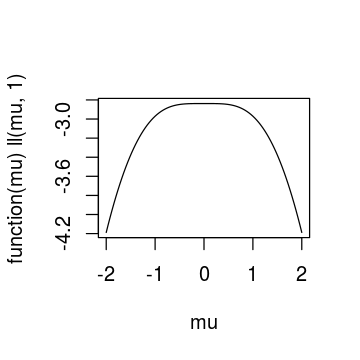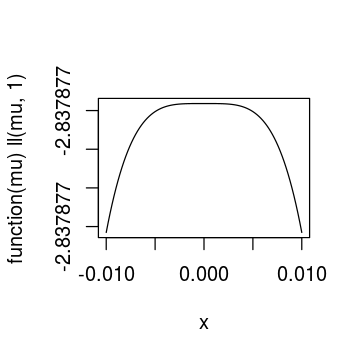# The Hessian¶

Pause for a moment to notice how flat the top of that graph is. Not only is this a critical point, but the Hessian is singular, so if we start near the critical point, even a second-order optimizer will be slow to get away. The Hessian is $\left( \begin{array}{cc}\frac{\partial^2}{\partial^2\mu} & \frac{\partial^2}{\partial\sigma\partial\mu} \\ \frac{\partial^2}{\partial\mu\partial\sigma} & \frac{\partial^2}{\partial^2\sigma}\end{array} \right)L = \left( \begin{array}{rr}0 & 0 \\ 0 & -4\end{array} \right)$:

In :
library(numDeriv)
hessian(function (x) ll(x,x), c(0,1))  # numerical Hessian
det(.Last.value)  # it's singular, obviously

1. 0
2. -9.99709819140423e-12
 -1.04952e-08 -2.64677e-09 -2.64677e-09 -4
4.19809200064392e-08

This really is a saddle point, not a local maximum, since there are better points arbitrarily close by (to find one, we have to nudge $\mu$ more than $\sigma$):

In :
ll(0.1,0.999) - ll(0,1)

1.31106506362499e-06
In :
ll(0.01,0.99999) - ll(0,1)

1.3331113990489e-10

It seems that alternating optimization led us right into the maw of a pathological case. Maybe gradient ascent will be better? There's no hope if we start with $\mu=0$ exactly -- we can't break the symmetry and we'll immediately jump to the saddle point $(0,1)$ and stay there. But as long as $\mu \neq 0$, we do prefer $\sigma < 1$. So the hope is that as long as we start at $\mu \neq 0$, then we'll eventually get to $\sigma < 1$, at which point $\mu$ will want to move away from 0 to specialize the clusters.

More precisely, the optimal $\sigma \neq 1$ for any $\mu \neq 0$. (You might expect that if $\mu=0.1$, then $\sigma=0.9$ so that the Gaussian at 0.1 can best explain the point at 1, but actually $\sigma > 0.9$ in this case so that it can help explain the point at -1 as well.)

Here are the optimal $\sigma$ values for $\mu=.1, .4, .6, .8, 1$. (The limiting case of $\mu=1$ is interesting because then the optimal clusters are Dirac deltas, i.e., $\sigma = 0$. For $\mu > 1$, which isn't shown here, the optimal $\sigma$ grows again ... in fact without bound as $\mu \rightarrow \infty$.)

In :
for (mu in c(.1,.4,.6,.8, 1)) plot(function (sigma) ll(mu,sigma), ylab="log lik", xlab="sigma", main=paste("mu=",mu), xlim=c(0.1,2))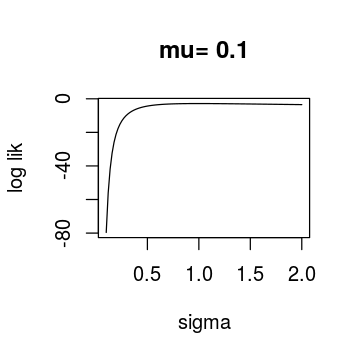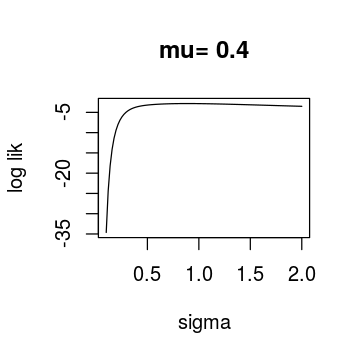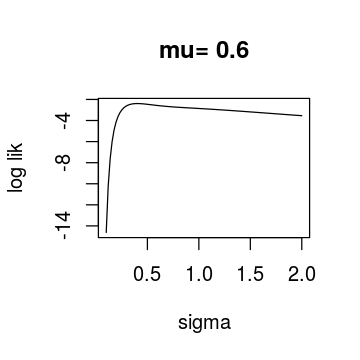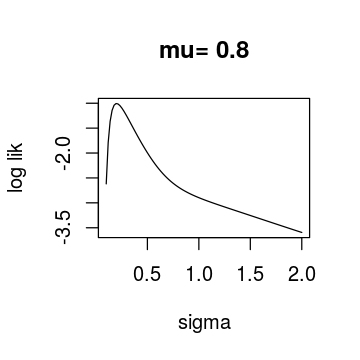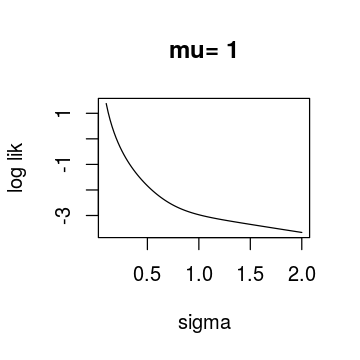Again, if we could keep $\mu \neq 0$ long enough to get to $\sigma < 1$, we'd be out of danger -- at least if $\sigma$ stayed $< 1$ -- since then the Gaussians would prefer to separate, leading us to the global max. To see the separation, here's what $\mu$ prefers for $\sigma=0.9$:

In :
plot(function (mu) ll(mu,.9), ylab="log lik", xlim=c(-2,2), xlab="mu")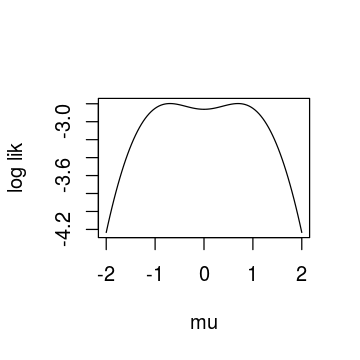This doesn't guarantee that we'll be saved from the saddle point. One might worry that if we start with $\sigma > 1$, which prefers $\mu = 0$, then $\mu$ might approach 0 so quickly that $\sigma$ doesn't have a chance to pull away from 0 and achieve "escape velocity." However, the plots below seem to show that this problem does not arise: $\sigma$ changes more quickly than $\mu$ if we follow the gradient.

In :
options(repr.plot.width=7, repr.plot.height=7)

In :
# Show the vector field of a function's gradients (in gray),
# as well as some trajectories followed by gradient ascent (in dark green with green arrowheads).
# The function must be from R^2 -> R.
# partial derivatives of fun (should probably use grad from numDeriv package instead, but that won't eval at many points in parallel)
# methods that will be used below to draw gradient steps
drawfield     <- function (x0,y0,x1,y1) { suppressWarnings(arrows(x0,y0,x1,y1, length=0.05, angle=15, col="gray65")) }
drawtraj      <- function (x0,y0,x1,y1) { segments(x0,y0,x1,y1, col="darkgreen") }   # line segments with no head
drawtrajlast  <- function (x0,y0,x1,y1) { suppressWarnings(arrows(x0,y0,x1,y1, length=0.1, col="green")) }
# grid of points
x <- seq(xlim,xlim,length.out=nn)
y <- seq(ylim,ylim,length.out=nn)
xx <- outer(x,y, function (x,y) x)
yy <- outer(x,y, function (x,y) y)
# draw the function using filled.contour, and imposed the arrows
filled.contour(x,y,fun(xx,yy),
plot.axes = {    # how to add to a filled.contour graph
axis(1); axis(2)    # label the axes
# draws a bunch of gradient steps in parallel starting at (xx,yy), and sets (newxx,newyy) to endpoints
segs <- function (xx,yy,scale,draw) { draw( xx, yy,
newyy <<- yy + scale*grady(xx,yy) ) }
# vector field
segs(xx,yy, scale,drawfield)
# grid of points where we'll start trajectories
seqmid <- function (lo,hi,len) { nudge <- (hi-lo)/(2*len); seq(lo+nudge,hi-nudge,length.out=len) }  # e.g., seqmid(0,1,5) gives 0.1,0.3,...,0.9, the same as the odd positions in seq(0,1,11)
x <- seqmid(xlim,xlim,nntraj)
y <- seqmid(ylim,ylim,nntraj)
xx <- outer(x,y, function (x,y) x)
yy <- outer(x,y, function (x,y) y)
# draw those trajectories
for (i in 1:iters) {
segs(xx,yy, scaletraj, if (i < iters) drawtraj else drawtrajlast)
done <- gradx(xx,yy)==0 & grady(xx,yy)==0      # which trajectories won't move any more?
xx <- ifelse(done, xx, newxx)  # update for next segment except where there wouldn't be a next segment
yy <- ifelse(done, yy, newyy)
}
# hack for this application: plot markers at specific critical points
points( c(0,1,-1),c(1,0,0),col=2)
})
}


In :
gradient_field(ll,c(-2,2),c(0.5,1.5), scale=0.03)